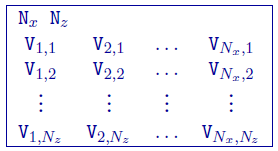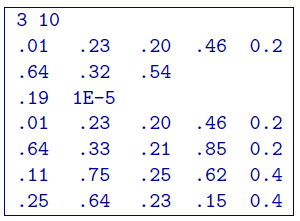# 5.4.2. 2D Model file¶

This is the file used to define any model given to . Each file contains the cell values within the model. The number of cells in the $$x-$$ and $$z-$$directions are specified in this file and should coorespond to the values given in the file.

An example of the file structure is as follows:• $$N_x$$: Number of cells in the horizontal direction

• $$N_z$$: Number of cells in the vertical direction.

• $$N_{i,k}$$: Value of the model at the i$$^{th}$$ cell in the horizontal

direction and k$$^{th}$$ cell in depth. When $$k=1$$, the cell is at the top of the mesh and $$k=N_z$$ corresponds to the bottom of the mesh. In the horizontal direction $$i=1$$ corresponds to the beginning of the line (e.g., West) and $$i=N_x$$ is at the end of the line (e.g., East).

• $$X_i$$: i$$^{th}$$ horizontal location

• $$elev_i$$: i$$^{th}$$ elevation

## 5.4.2.1. Example¶

The following is an example of a model:In the above example, there are 10 horizontal cells and 3 vertical cell associated with the mesh. The first row is broken into the first three lines of the file. The second row of the model is given on lines 2-4. The third row of the model is given on lines 5 and 6. would also read the same model with three rows of ten values.

## 5.4.2.2. DC/IP 2D notes¶

The model is stored in a row format with V$$_{1,1}$$ being the top left cell. As shown above, each row of the model, $$V_{1,k} … V_{N_x,k}$$ , can be broken down into more than a single line in the file, however, each model row must start on a new line.

In the presence of topography, the cells above the surface specified by topo.dat represent the air and, therefore, are not part of the model. For simplicity, however, the files model.con and model.chg must include those cells as defined by the file. These cells in the model file will be ignored when the model is input to a program. To distinguish these cells, the user can assign a special value to them, for instance, a very small value (say 10$$^{-10}$$) for conductivity and a negative value for the chargeability model. These cells in the output model files from the inversion programs have a conductivity value equal to 10$$^{-8}$$ times the mean conductivity value immediately beneath the surface and a chargeability value of -10$$^{30}$$.

2D active model file

The active cell model file is a specific type of model that has the same format, but only contains values of -1, 0, or 1. Default ( option) active cells models place all cells in the model as active, meaning they can be changed during the inversion and influence the model objective function and data misfit. Cells that are inactive (not allowed to change) and do not influence the model objective function should be set to 0. Cells that are inactive, but can influence the values of neighbouring cells through the model objective function should have a value of -1.